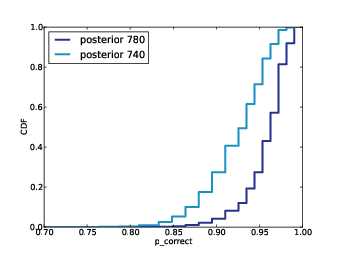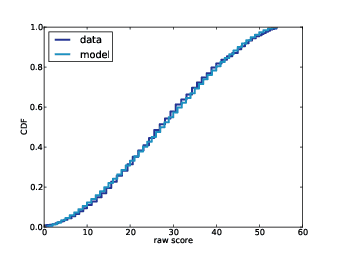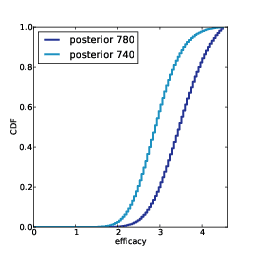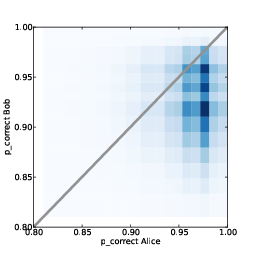## Friday, February 8, 2013

### Belly Button Biodiversity: Part Two

This is part two of a series of articles about a Bayesian solution to the Unseen Species problem, applied to data from the Belly Button Biodiversity project.

In Part One I presented the simplest version of the algorithm, which I think is easy to understand, but slow.  In Think Bayes I present some ways to optimize it.  Now in Part Two I apply the algorithm to real data and generate predictive distributions.  In Part Three I will publish the predictions the algorithm generates, and in Part Four I will compare the predictions to actual data.

Background: Belly Button Biodiversity 2.0 (BBB2) is a nation-wide citizen science project with the goal of identifying bacterial species that can be found in human navels (http://bbdata.yourwildlife.org).

### The belly button data

To get a sense of what the data look like, consider subject B1242, whose sample of 400 reads yielded 61 species with the following counts:
```92, 53, 47, 38, 15, 14, 12, 10, 8, 7, 7, 5, 5,
4, 4, 4, 4, 4, 4, 4, 3, 3, 3, 3, 3, 3, 3, 2, 2, 2, 2,
1, 1, 1, 1, 1, 1, 1, 1, 1, 1, 1, 1, 1, 1, 1, 1, 1, 1,
1, 1, 1, 1, 1, 1, 1, 1, 1, 1, 1, 1
```
There are a few dominant species that make up a substantial fraction of the whole, but many species that yielded only a single read. The number of these “singletons” suggests that there are likely to be at least a few unseen species.
In the example with lions and tigers, we assume that each animal in the preserve is equally likely to be observed. Similarly, for the belly button data, we assume that each bacterium is equally likely to yield a read.
In reality, it is possible that each step in the data-collection process might introduce consistent biases. Some species might be more likely to be picked up by a swab, or to yield identifiable amplicons. So when we talk about the prevalence of each species, we should remember this source of error.
I should also acknowledge that I am using the term “species” loosely. First, bacterial species are not well-defined. Second, some reads identify a particular species, others only identify a genus. To be more precise, I should say “operational taxonomic unit”, or OTU.
Now let’s process some of the belly button data. I defined a class called Subject to represent information about each subject in the study:
```class Subject(object):

def __init__(self, code):
self.code = code
self.species = []
```
Each subject has a string code, like “B1242”, and a list of (count, species name) pairs, sorted in increasing order by count. Subject provides several methods to make it easy to these counts and species names. You can see the details in http://thinkbayes.com/species.py.Figure 12.3: Distribution of n for subject B1242.

In addition, Subject.Process creates a suite, specifically a suite of type Species5, which represents the distribution of n and the prevalences after processing the data.
It also provides PlotDistOfN, which plots the posterior distribution of n. Figure 12.3 shows this distribution for subject B1242. The probability that there are exactly 61 species, and no unseen species, is nearly zero. The most likely value is 72, with 90% credible interval 66 to 79. At the high end, it is unlikely that there are as many as 87 species.
Next we compute the posterior distribution of prevalence for each species. Species2 providesDistOfPrevalence:
```# class Species2

def DistOfPrevalence(self, index):
pmfs = thinkbayes.Pmf()

for n, prob in zip(self.ns, self.probs):
beta = self.MarginalBeta(n, index)
pmf = beta.MakePmf()
pmfs.Set(pmf, prob)

mix = thinkbayes.MakeMixture(pmfs)
return pmfs, mix
```
index indicates which species we want. For each value of n, we have a different posterior distribution of prevalence.Figure 12.4: Distribution of prevalences for subject B1242.

So the loop iterates through the possible values of n and their probabilities. For each value of n it gets a Beta object representing the marginal distribution for the indicated species. Remember that Beta objects contain the parameters alpha and beta; they don’t have values and probabilities like a Pmf, but they provide MakePmf which generates a discrete approximation to the continuous beta distribution.
pmfs is a MetaPmf that contains the distributions of prevalence, conditioned on nMakeMixturecombines the MetaPmf into mix, which combines the conditional distributions into the answer, a single distribution of prevalence.
Figure 12.4 shows these distributions for the five species with the most reads. The most prevalent species accounts for 23% of the 400 reads, but since there are almost certainly unseen species, the most likely estimate for its prevalence is 20%, with 90% credible interval between 17% and 23%.

### Predictive distributionsFigure 12.5: Simulated rarefaction curves for subject B1242.

I introduced the hidden species problem in the form of four related questions. We have answered the first two by computing the posterior distribution for n and the prevalence of each species.
The other two questions are:
• If we are planning to collect additional samples, can we predict how many new species we are likely to discover?
• How many additional reads are needed to increase the fraction of observed species to a given threshold?
To answer predictive questions like this we can use the posterior distributions to simulate possible future events and compute predictive distributions for the number of species, and fraction of the total, we are likely to see.
The kernel of these simulations looks like this:
1. Choose n from its posterior distribution.
2. Choose a prevalence for each species, including possible unseen species, using the Dirichlet distribution.
3. Generate a random sequence of future observations.
4. Compute the number of new species, `num_new`, as a function of the number of additional samples, k.
5. Repeat the previous steps and accumulate the joint distribution of `num_new` and k.
And here’s the code. RunSimulation runs a single simulation:
```# class Subject

def RunSimulation(self, num_samples):
m, seen = self.GetSeenSpecies()
n, observations = self.GenerateObservations(num_samples)

curve = []
for k, obs in enumerate(observations):

num_new = len(seen) - m
curve.append((k+1, num_new))

return curve
```
`num_samples` is the number of additional samples to simulate. m is the number of seen species, and seen is a set of strings with a unique name for each species. n is a random value from the posterior distribution, and observations is a random sequence of species names.
The result of RunSimulation is a “rarefaction curve”, represented as a list of pairs with the number of samples and the number of new species seen.
Before we see the results, let’s look at GetSeenSpecies and GenerateObservations.
```#class Subject

def GetSeenSpecies(self):
names = self.GetNames()
m = len(names)
seen = set(SpeciesGenerator(names, m))
return m, seen
```
GetNames returns the list of species names that appear in the data files, but for many subjects these names are not unique. So I use SpeciesGenerator to extend each name with a serial number:
```def SpeciesGenerator(names, num):
i = 0
for name in names:
yield '%s-%d' % (name, i)
i += 1

while i < num:
yield 'unseen-%d' % i
i += 1
```
Given a name like CorynebacteriumSpeciesGenerator yields Corynebacterium-1. When the list of names is exhausted, it yields names like unseen-62.
Here is GenerateObservations:
```# class Subject

def GenerateObservations(self, num_samples):
n, prevalences = self.suite.Sample()

names = self.GetNames()
name_iter = SpeciesGenerator(names, n)

d = dict(zip(name_iter, prevalences))
cdf = thinkbayes.MakeCdfFromDict(d)
observations = cdf.Sample(num_samples)

return n, observations
```
Again, `num_samples` is the number of additional samples to generate. n and prevalences are samples from the posterior distribution.
cdf is a Cdf object that maps species names, including the unseen, to cumulative probabilities. Using a Cdf makes it efficient to generate a random sequence of species names.
Finally, here is Species2.Sample:
```    def Sample(self):
pmf = self.DistOfN()
n = pmf.Random()
prevalences = self.SampleConditional(n)
return n, prevalences
```
And SampleConditional, which generates a sample of prevalences conditioned on n:
```# class Species2

def SampleConditional(self, n):
params = self.params[:n]
gammas = numpy.random.gamma(params)
gammas /= gammas.sum()
return gammas
```
We saw this algorithm for generating prevalences previously in Species2.SampleLikelihood.
Figure 12.5 shows 100 simulated rarefaction curves for subject B1242. I shifted each curve by a random offset so they would not all overlap. By inspection we can estimate that after 400 more samples we are likely to find 2–6 new species.

### Joint posteriorFigure 12.6: Distributions of the number of new species conditioned on the number of additional samples.

To be more precise, we can use the simulations to estimate the joint distribution of `num_new` andk, and from that we can get the distribution of `num_new` conditioned on any value of k.
```# class Subject

def MakeJointPredictive(self, curves):
joint = thinkbayes.Joint()
for curve in curves:
for k, num_new in curve:
joint.Incr((k, num_new))
joint.Normalize()
return joint
```
MakeJointPredictive makes a Joint object, which is a Pmf whose values are tuples.
curves is a list of rarefaction curves created by RunSimulation. Each curve contains a list of pairs of k and `num_new`.
The resulting joint distribution is a map from each pair to its probability of occurring. Given the joint distribution, we can get the distribution of `num_new` conditioned on k:
```# class Joint

def Conditional(self, i, j, val):
pmf = Pmf()
for vs, prob in self.Items():
if vs[j] != val: continue
pmf.Incr(vs[i], prob)

pmf.Normalize()
return pmf
```
i is the index of the variable whose distribution we want; j is the index of the conditional variables, and val is the value the jth variable has to have. You can think of this operation as taking vertical slices out of Figure 12.5.
Subject.MakeConditionals takes a list of ks and computes the conditional distribution of `num_new` for each k. The result is a list of Cdf objects.
```# class Subject

def MakeConditionals(self, curves, ks):
joint = self.MakeJointPredictive(curves)

cdfs = []
for k in ks:
pmf = joint.Conditional(1, 0, k)
pmf.name = 'k=%d' % k
cdf = thinkbayes.MakeCdfFromPmf(pmf)
cdfs.append(cdf)

return cdfs
```
Figure 12.6 shows the results. After 100 samples, the median predicted number of new species is 2; the 90% credible interval is 0 to 5. After 800 samples, we expect to see 3 to 12 new species.

### CoverageFigure 12.7: Complementary CDF of coverage for a range of additional samples.

The last question we want to answer is, “How many additional reads are needed to increase the fraction of observed species to a given threshold?”
To answer this question, we’ll need a version of RunSimulation that computes the fraction of observed species rather than the number of new species.
```# class Subject

def RunSimulation(self, num_samples):
m, seen = self.GetSeenSpecies()
n, observations = self.GenerateObservations(num_samples)

curve = []
for k, obs in enumerate(observations):

frac_seen = len(seen) / float(n)
curve.append((k+1, frac_seen))

return curve
```
Next we loop through each curve and make a dictionary, d, that maps from the number of additional samples, k, to a list of fracs; that is, a list of values for the coverage achieved after ksamples.
```    def MakeFracCdfs(self, curves):
d = {}
for curve in curves:
for k, frac in curve:
d.setdefault(k, []).append(frac)

cdfs = {}
for k, fracs in d.iteritems():
cdf = thinkbayes.MakeCdfFromList(fracs)
cdfs[k] = cdf

return cdfs
```
Then for each value of k we make a Cdf of fracs; this Cdf represents the distribution of coverage after k samples.
Remember that the CDF tells you the probability of falling below a given threshold, so thecomplementary CDF tells you the probability of exceeding it. Figure 12.7 shows complementary CDFs for a range of values of k.
To read this figure, select the level of coverage you want to achieve along the x-axis. As an example, choose 90%.
Now you can read up the chart to find the probability of achieving 90% coverage after k samples. For example, with 300 samples, you have about a 60% of getting 90% coverage. With 700 samples, you have a 90% chance of getting 90% coverage.
With that, we have answered the four questions that make up the unseen species problem. Next time: validation!